Courses

# NCERT Exemplar - Coordinate Geometry Class 10 Notes | EduRev

## Class 10 : NCERT Exemplar - Coordinate Geometry Class 10 Notes | EduRev

The document NCERT Exemplar - Coordinate Geometry Class 10 Notes | EduRev is a part of the Class 10 Course Mathematics (Maths) Class 10.
All you need of Class 10 at this link: Class 10

Q.1. AOBC is a rectangle whose three vertices are A (0, 3), O (0, 0) and B (5, 0). Find the length of its diagonal.
Ans.
Length of diagonal = AB =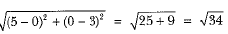Q.2. Find distance between the points (0, 5) and (- 5, 0).
Ans.
Here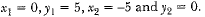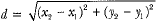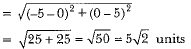Q.3. Show that ΔABC with vertices A(-2, 0), B(0, 2) and C(2, 0) is similar to ΔDEF with vertices D(-4, 0), F(4, 0) and E(0, 4).
[ΔPQR is replaced by ΔDEF]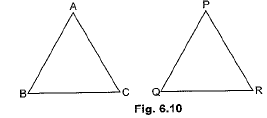Ans.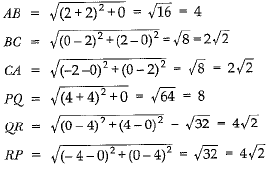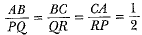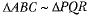Q.4. Find the values of ft if the points A (k + 1, 2k), B(3k, 2k + 3) and C(5k - 1, 5k) are collinear.
Ans.
∵ Points A(k + 1, 2k), B (3k, 2k + 3) and C (5k - 1, 5k) are collinear.
∴ Area of ΔABC = 0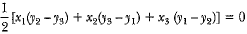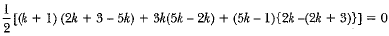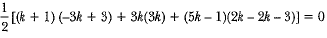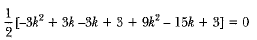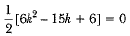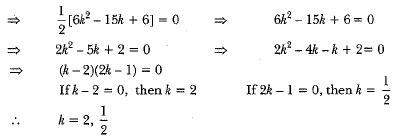Q.5. If the point A (2, -4) is equidistant from P (3, 8) and Q (-10, 3)), find the values of y. Also find distance PQ.
Ans.
Given points are A (2, -4), P(3, 8) and Q(—10, y)
According to the question,
PA = QA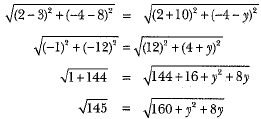On squaring both sides, we get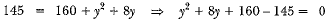y2 + 8y + 15 = 0
y2 + 5y + 3y + 15 = 0
⇒ y(y + 5) + 3(y + 5) = 0
⇒ (y+ 5) (y + 3) = 0
⇒  y + 5 = 0
⇒ y = -5
and y + 3 = 0
⇒ y = -3
∴ y =  -3, -5
Now,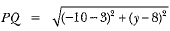For y = -3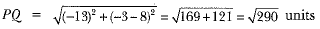and for y = -5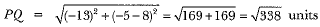Hence, values of y are - 3 and -5,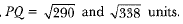Q.6. A (6, 1), B (8, 2) and C (9, 4) are three vertices of a parallelogram ABCD. If E is the mid-point of DC, find the area of ΔADE.
Ans.
Let (x, y) be coordinates of D.
Since we know that diagonals of a parallogram bisect each other.
So, mid-point of AC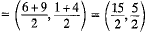Mid-point of BD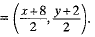Mid-point of AC and BD are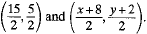By comparison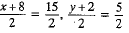x = 7, y = 3
Coordinates of D are (7, 3).
and coordinates of E are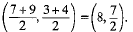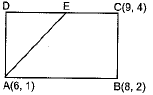Area of ΔADE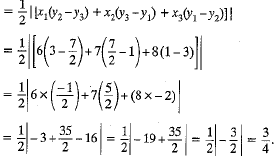Q.7. The points A (x1, y1), B (x2, y2) and C (x3, y3) are the vertices of ΔABC.
(i) The-median from A meets BC at D. Find the coordinates of the point D.
(ii) Find the coordinates of the point P on AD such that AP : PD = 2 : 1.
(iii) Find the coordinates of points Q and R on medians BE and CF, respectively such that BQ : QE = 2 : 1 and CR : RF = 2 : 1.
(iv) What are the coordinates of the centroid of the triangle ABC?
Ans.
(i) D is mid-point of BC.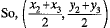(ii) If P is point on AD, then by section formula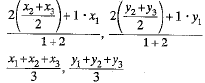(iii) Same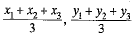∴ Its centroid divides all the medians in the ratio 2 : 1
(iv) Coordinates of the centroid are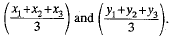Offer running on EduRev: Apply code STAYHOME200 to get INR 200 off on our premium plan EduRev Infinity!

## Mathematics (Maths) Class 10

51 videos|346 docs|103 tests

,

,

,

,

,

,

,

,

,

,

,

,

,

,

,

,

,

,

,

,

,

;